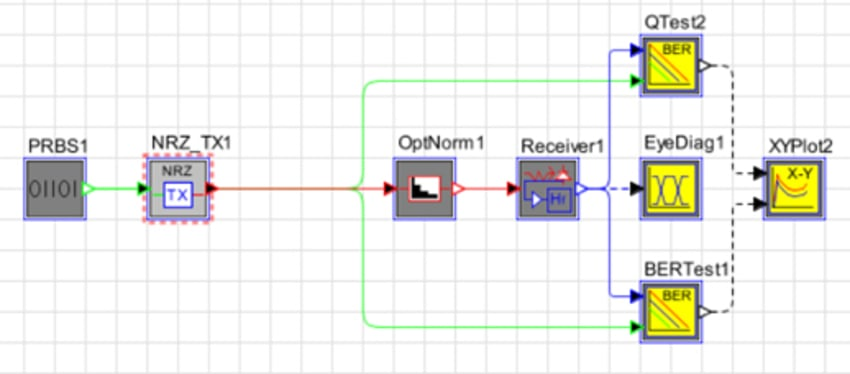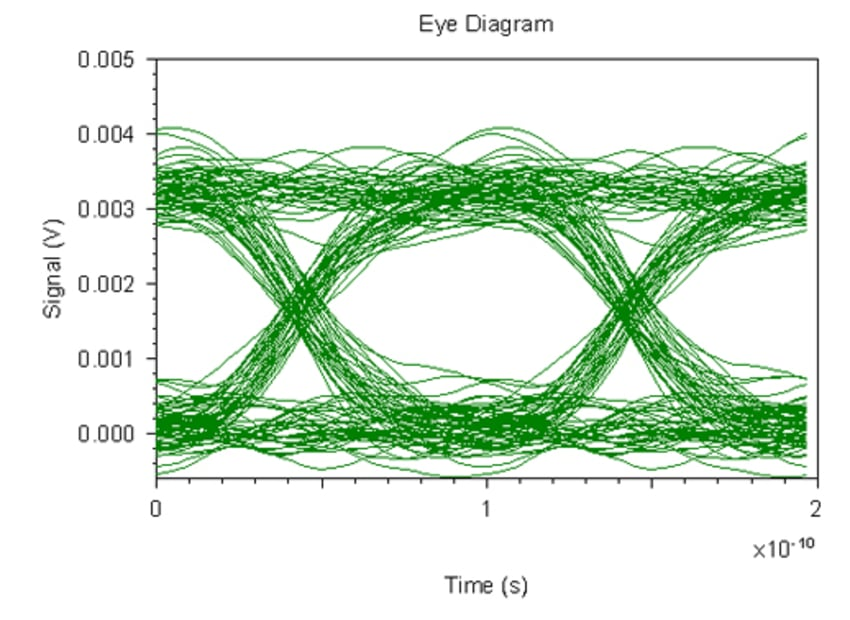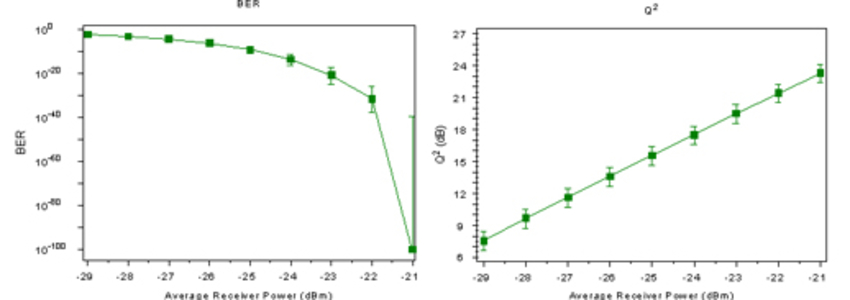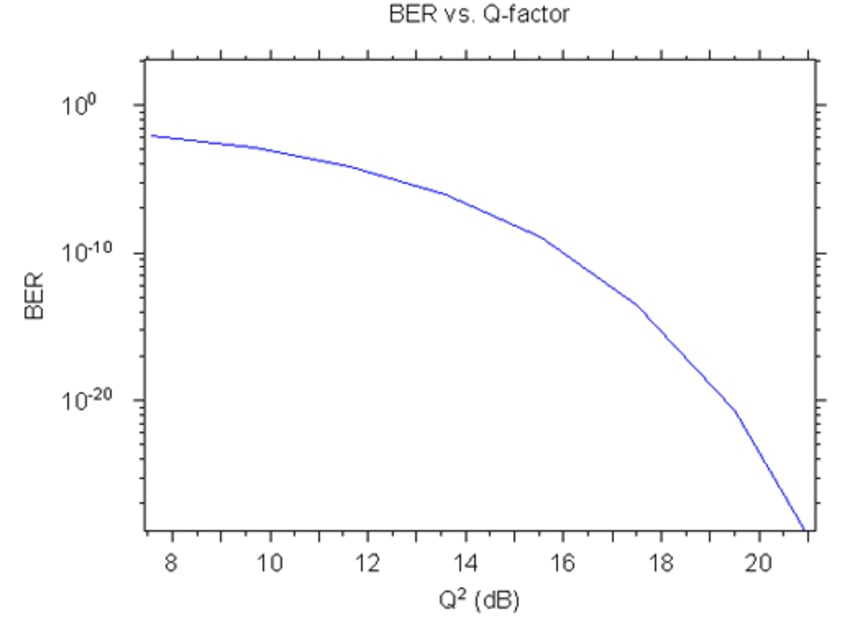# Receiver Sensitivity

## Tools Used: OptSim

The purpose of this example is to study the effect of optical receiver characteristics on a system performance.

The figure below depicts the layout of such a system in OptSim, consisting of: Bit Pattern Generator, NRZ Transmitter, Optical Power Normalizer, Optical Receiver, BER Tester, Eye Diagram Analyzer, and XY-Plotter.This sensitivity was achieved by adjusting the receiver parameters. Let us describe in details the receiver setting. Optical Receiver Model is a combination of a few components: PIN Photodiode, Electrical Filter, and electrical transimpedance amplifier (TIA). Photodiode is characterized by Responsivity, which is defined by quantum efficiency. Current at photodiode is derived as:

I(t) = R P(t) + N(t), where R is Responsivity, P(t) is the input optical signal, and N(t) is the noise generated at receiver. The current passed to TIA, converted to voltage, and sent passes through electrical filter. For electrical filter we used low- pass 4-th order Bessel with bandwidth 7.5 GHz.

Total noise is a combination of different noise contribution mechanisms: thermal noise from TIA, signal and dark current shot noise, RIN noise (depends of RIN value of optical sources such as lasers), signal-spontaneous and spontaneous-spontaneous beat noise. The receiver model allows to turn ON/OFF each of these noise contributions separately.

The figure below shows corresponding eye diagram at receiver for input power -25 dBm.Next we want to observe the effect of changing of receiver input power on BER. The parameter sweep for input power is from -29 to -21 dBm, i.e. within ±4 dB range from original input power.The plot of BER vs. Q-factor is shown below: